Successfully reported this slideshow.
We use your LinkedIn profile and activity data to personalize ads and to show you more relevant ads. You can change your ad preferences anytime.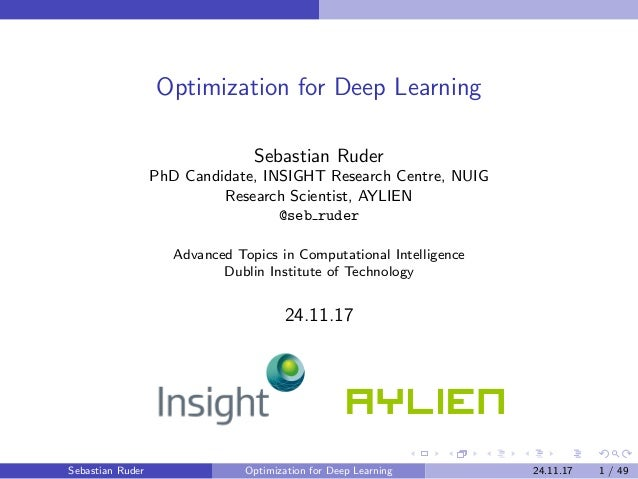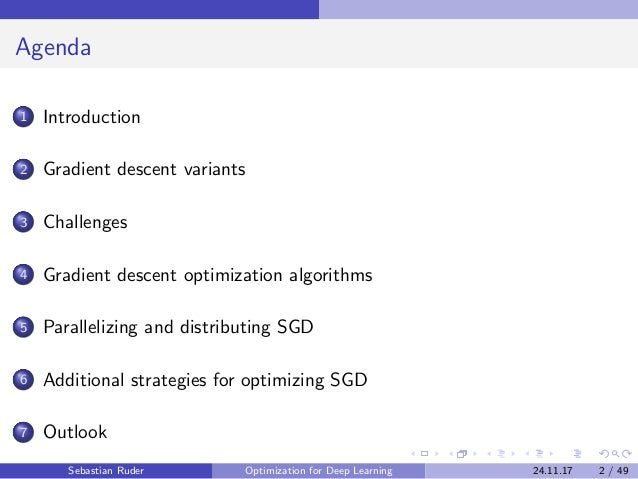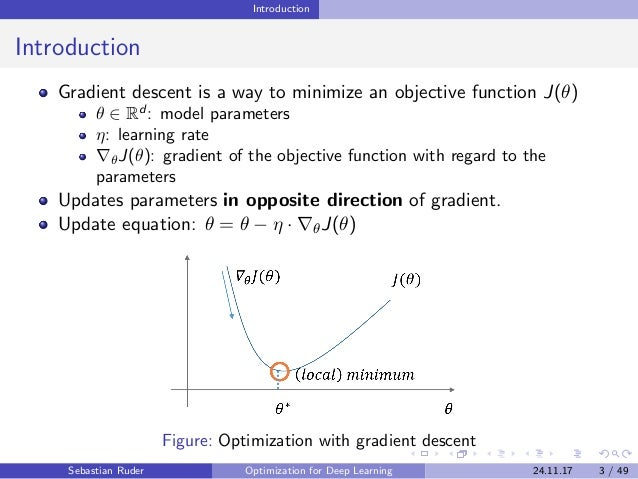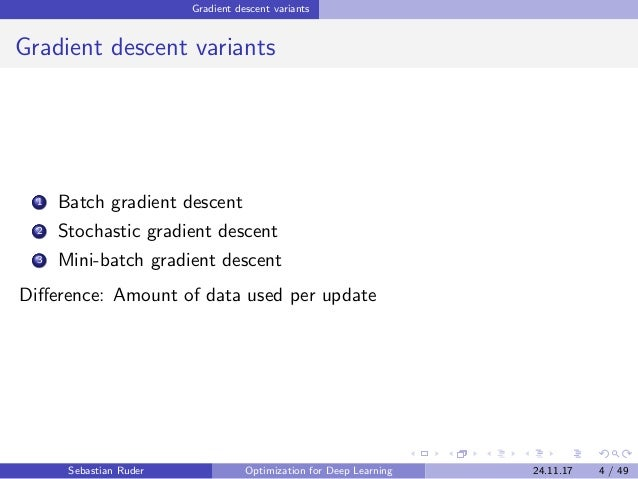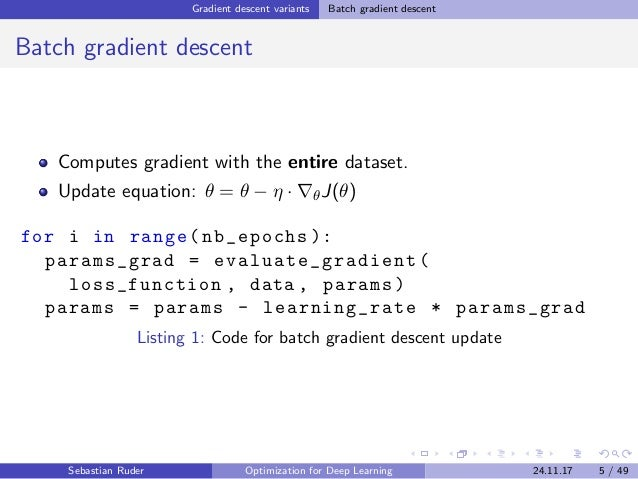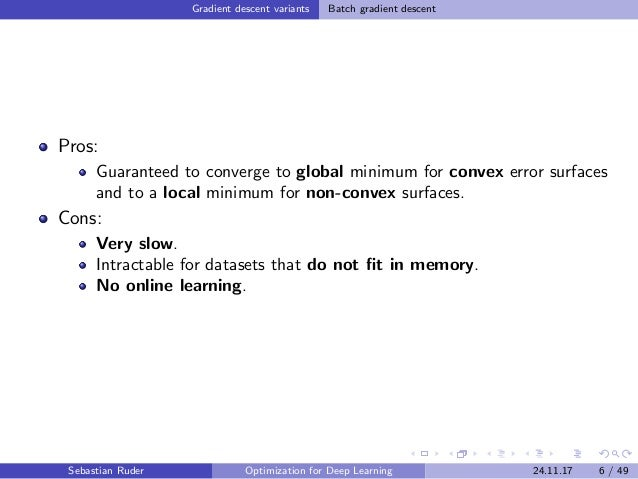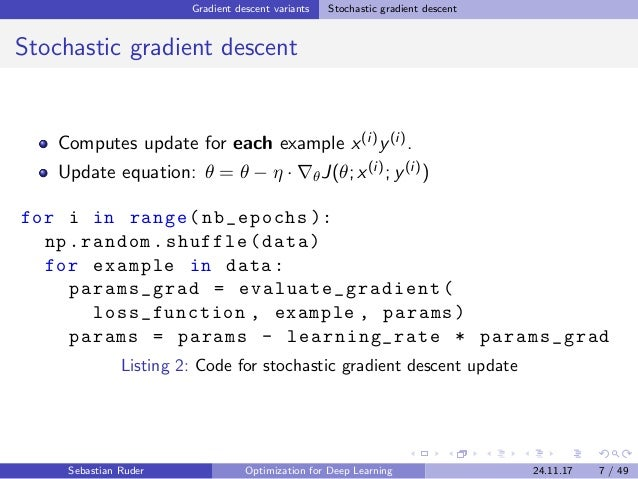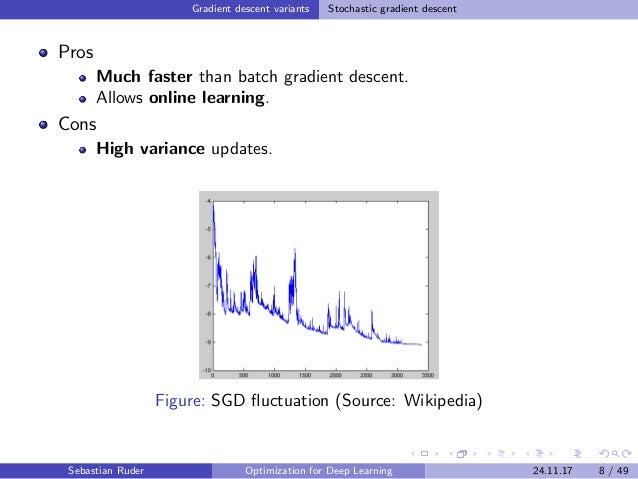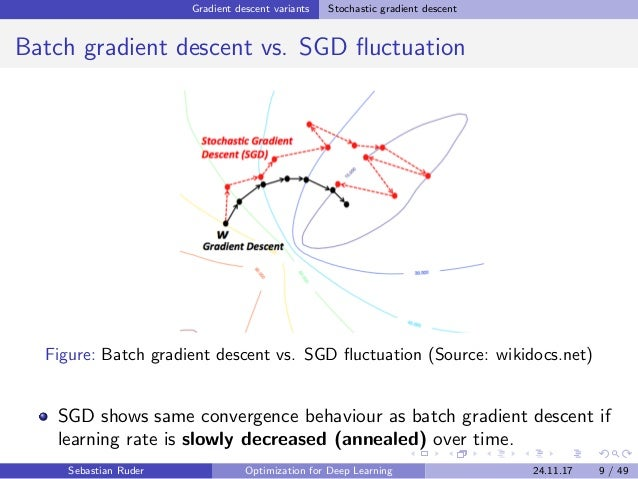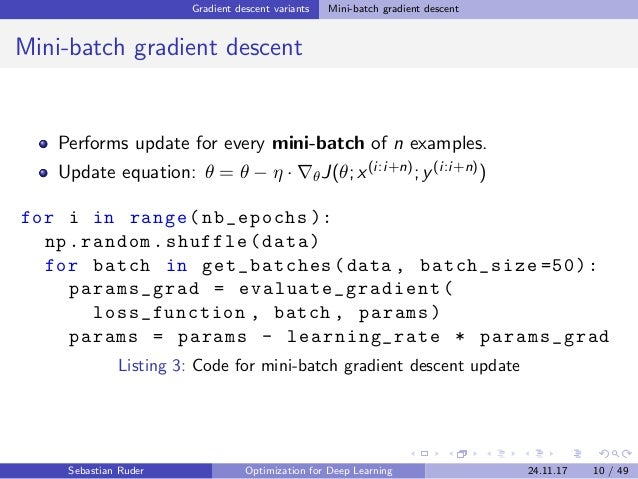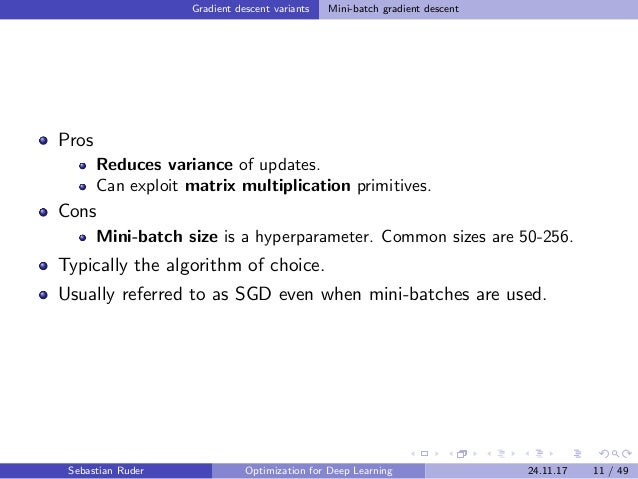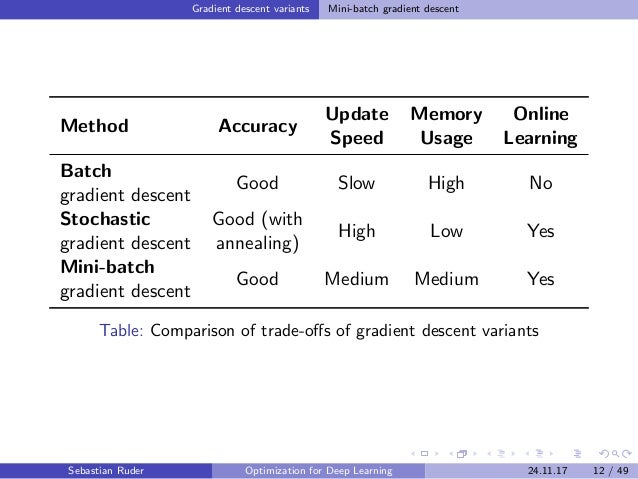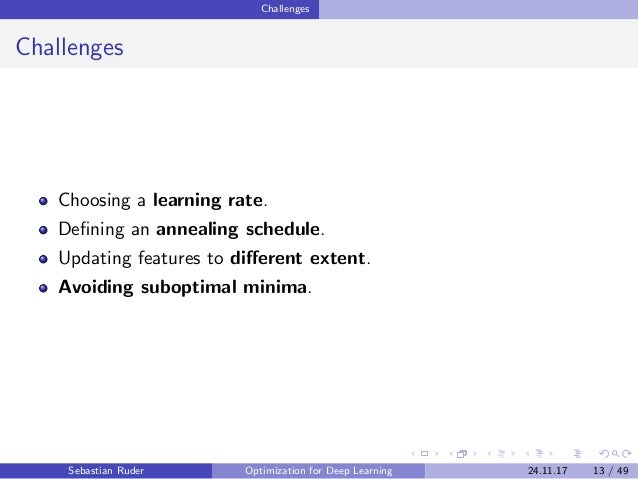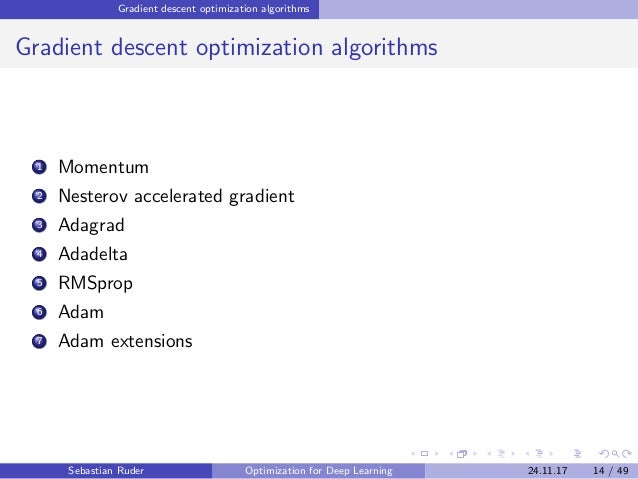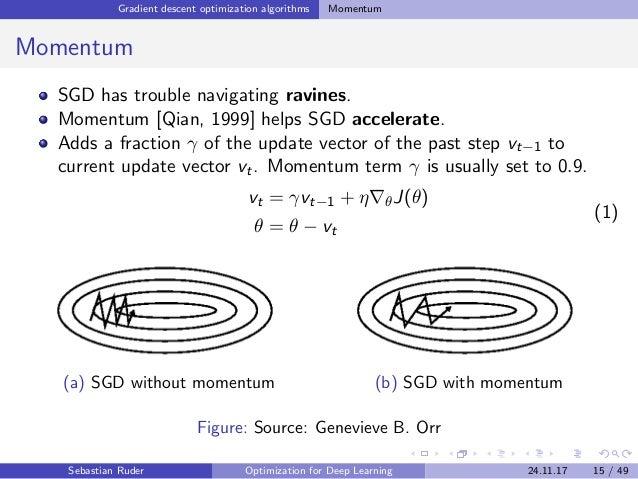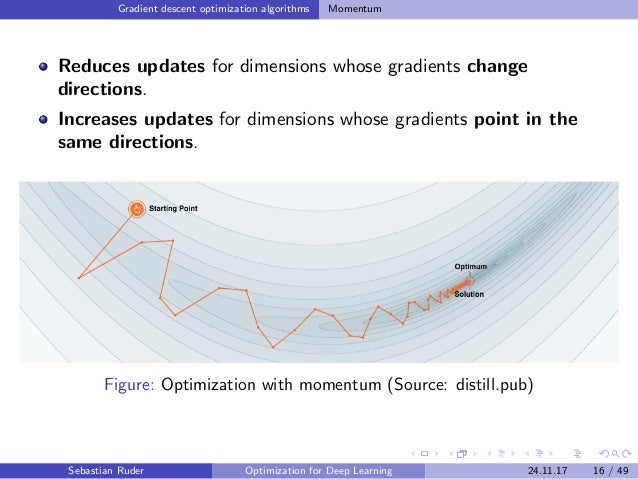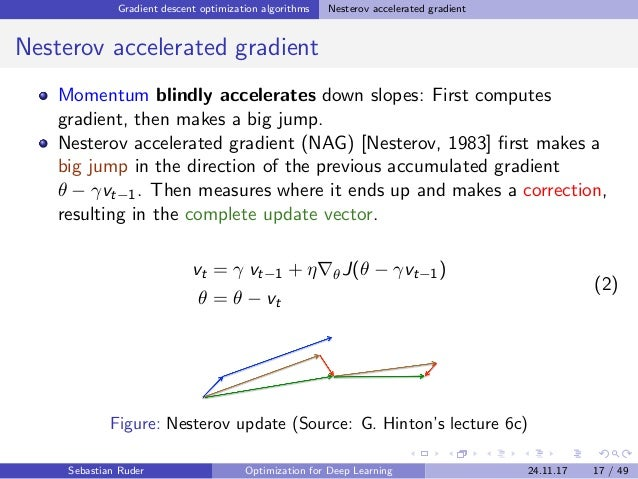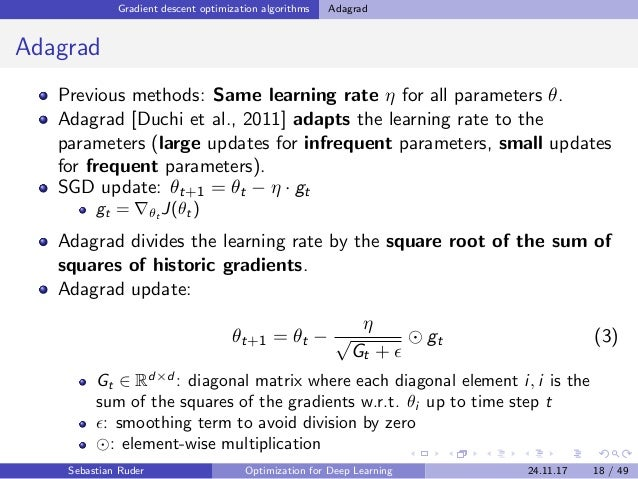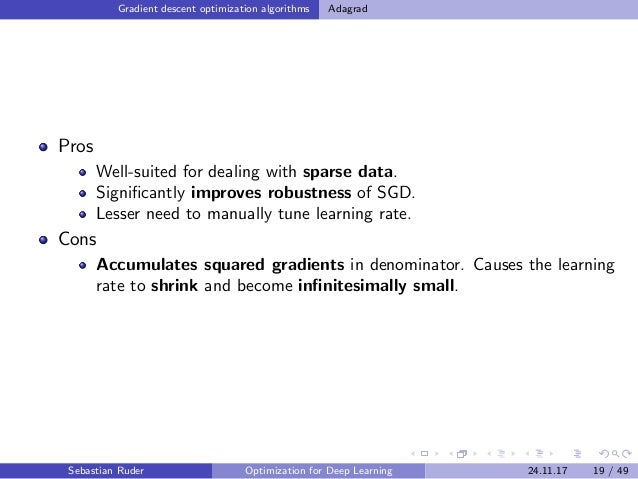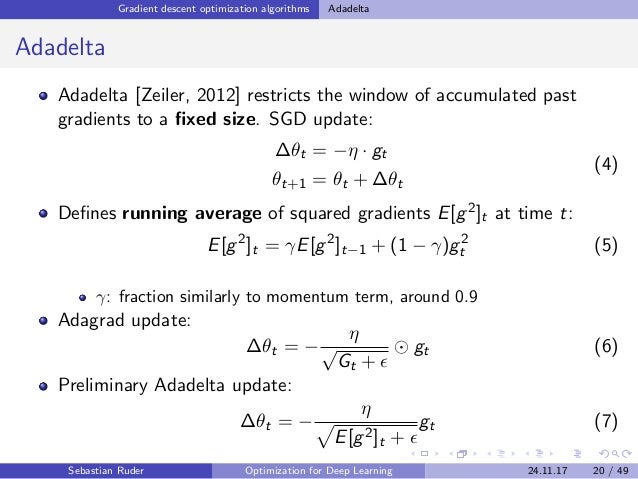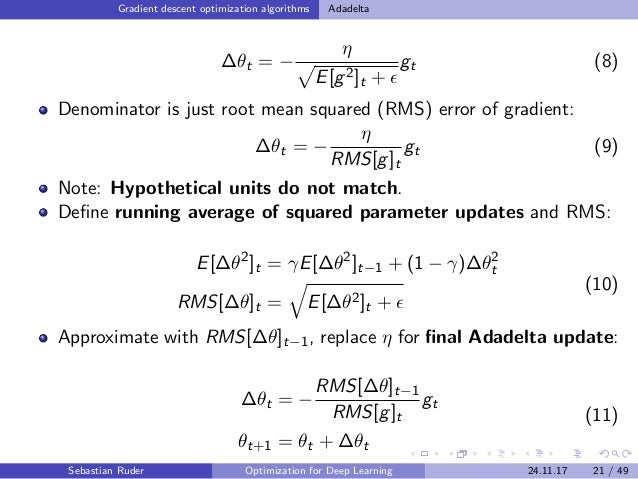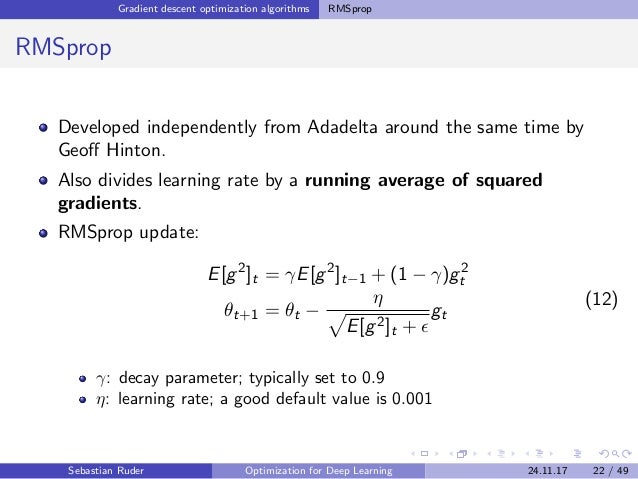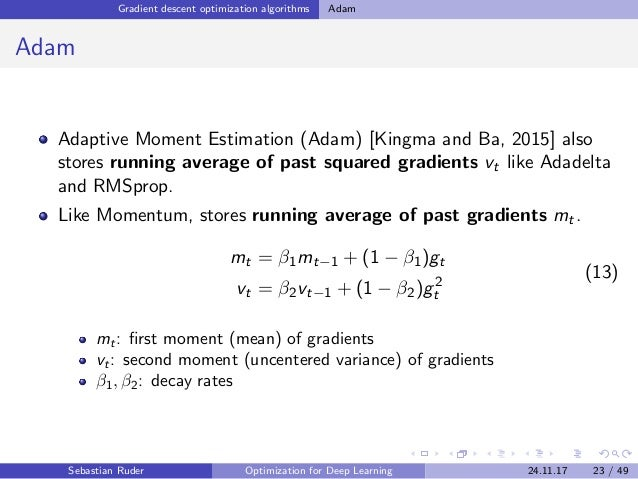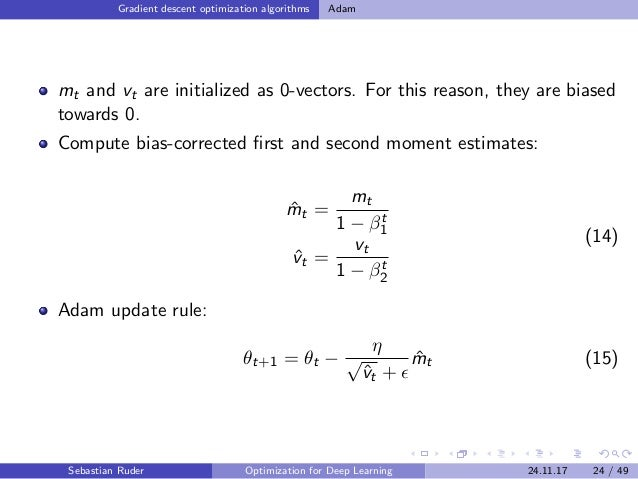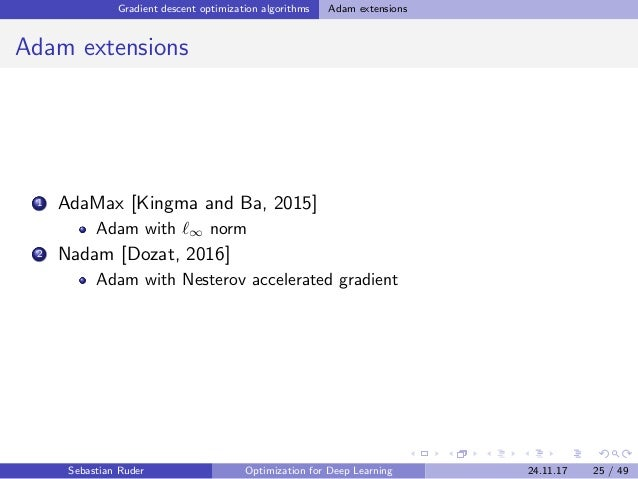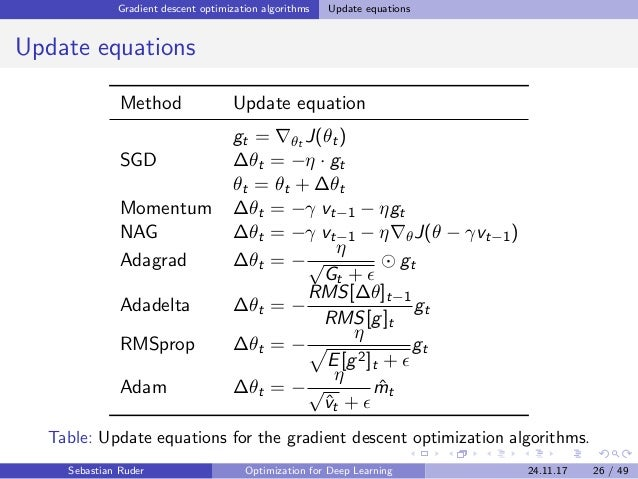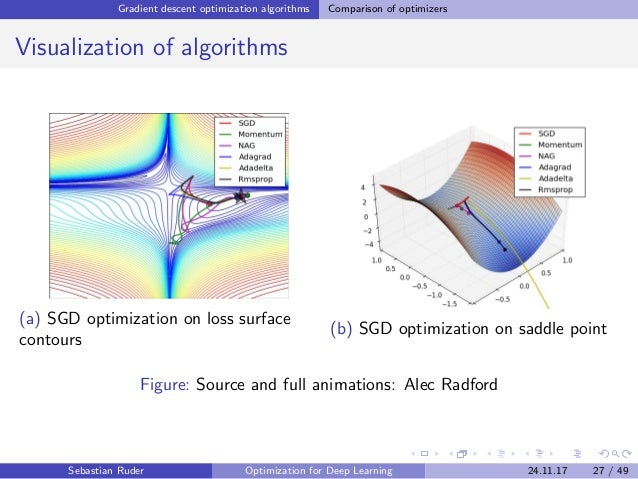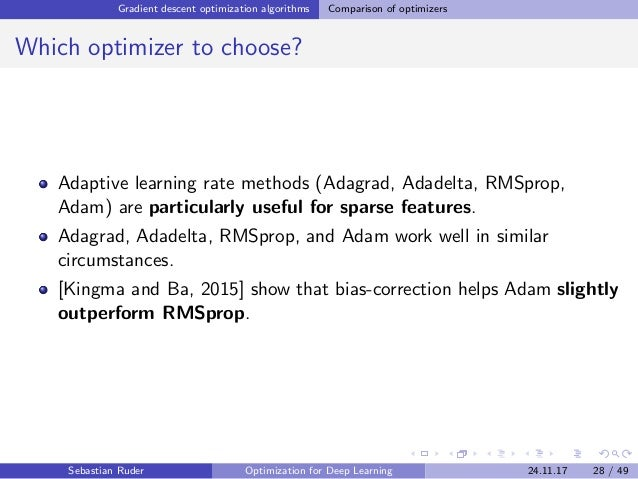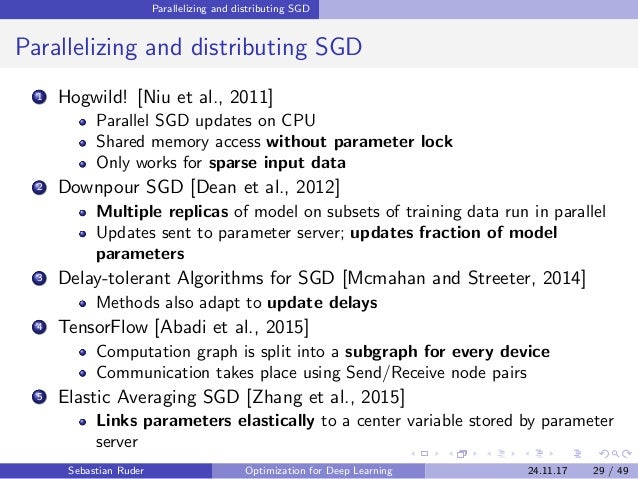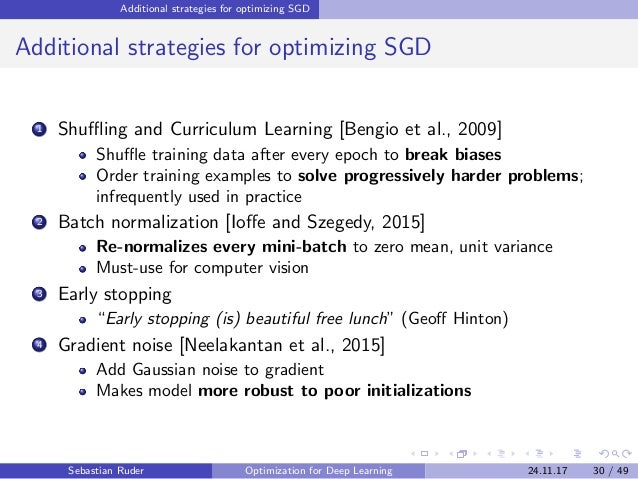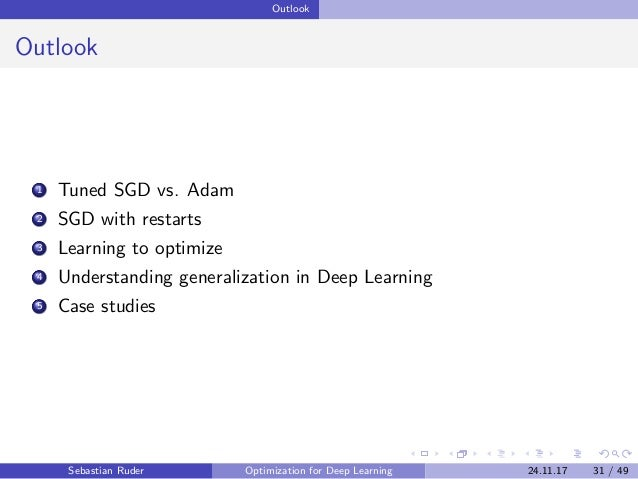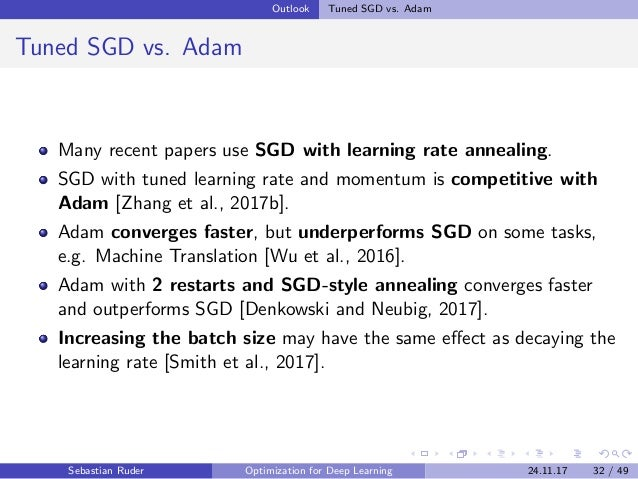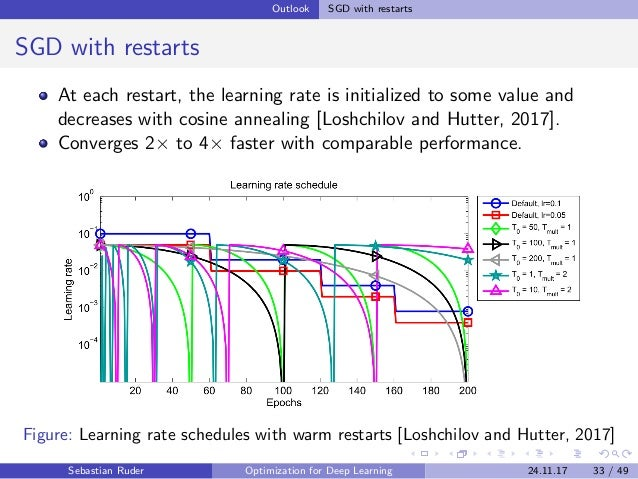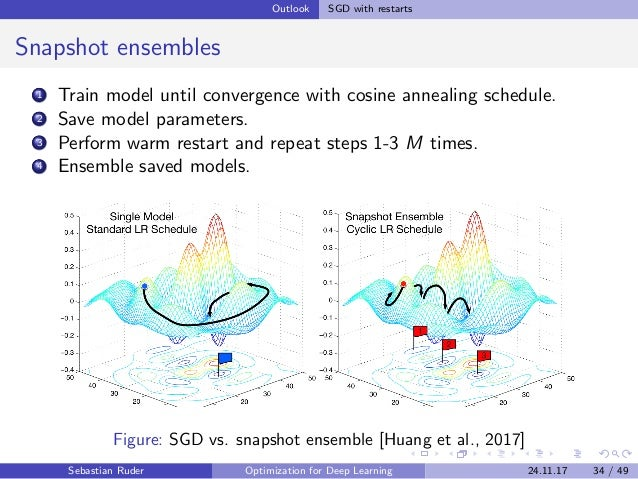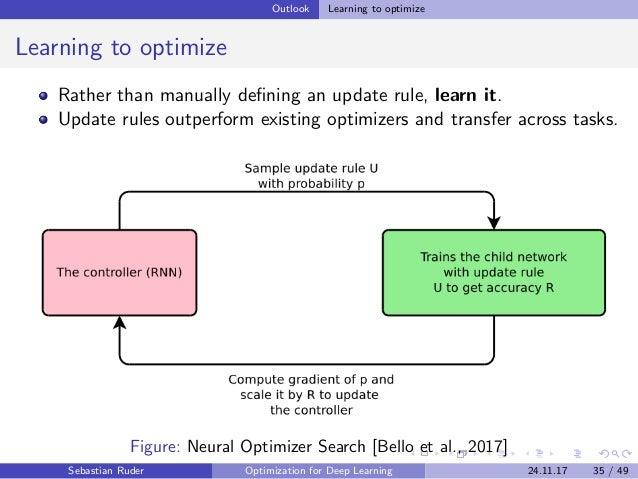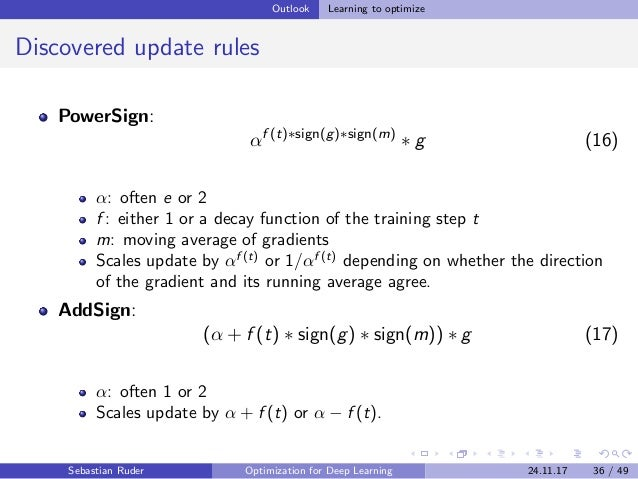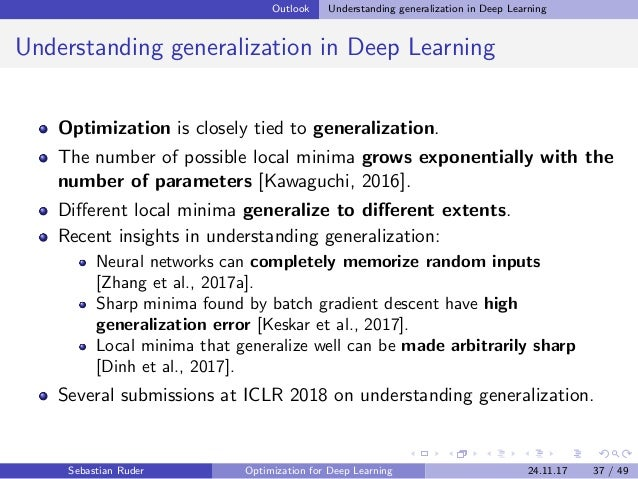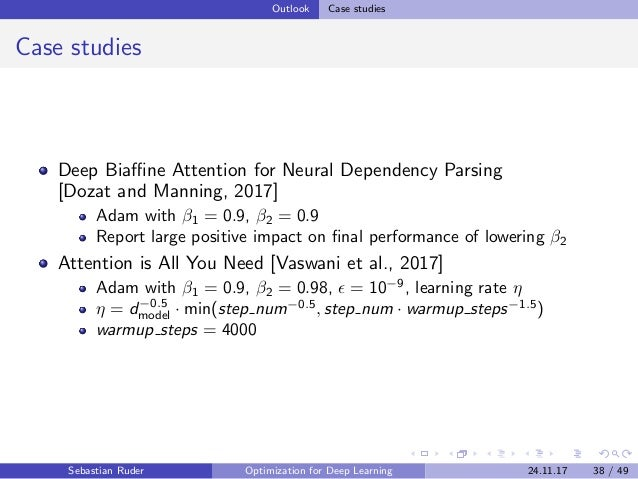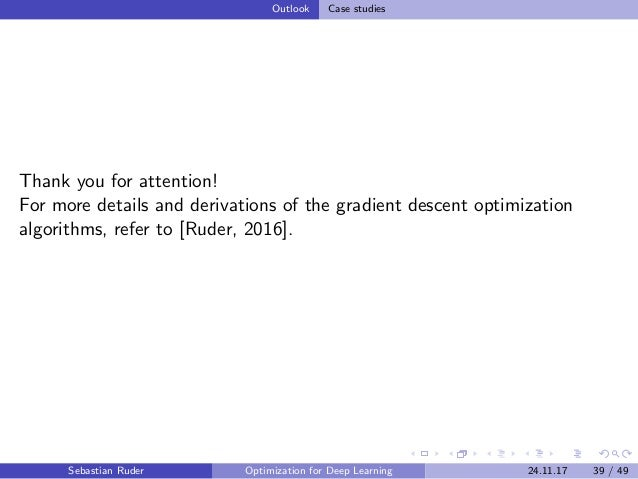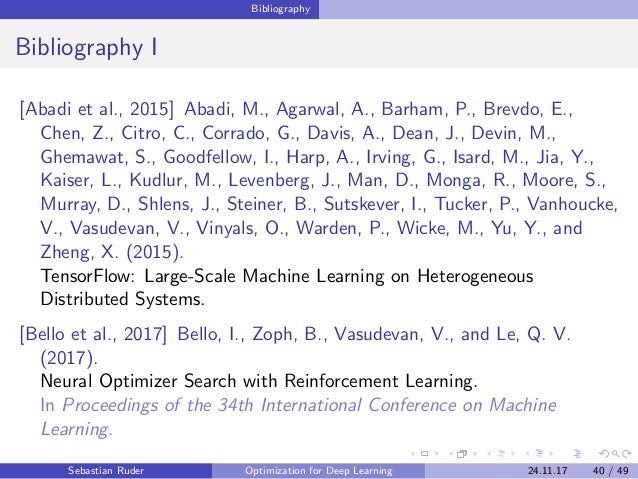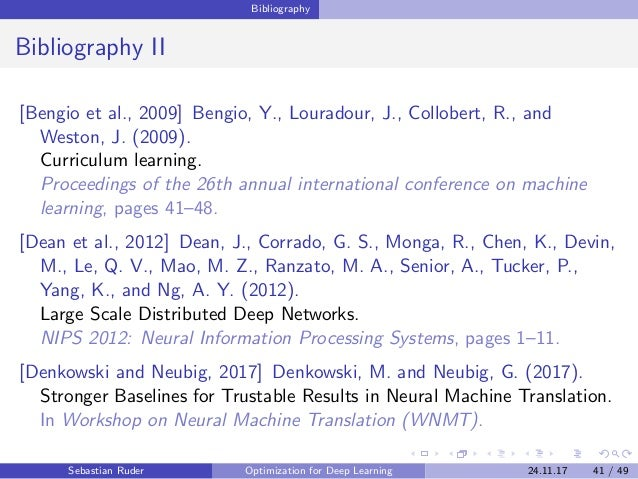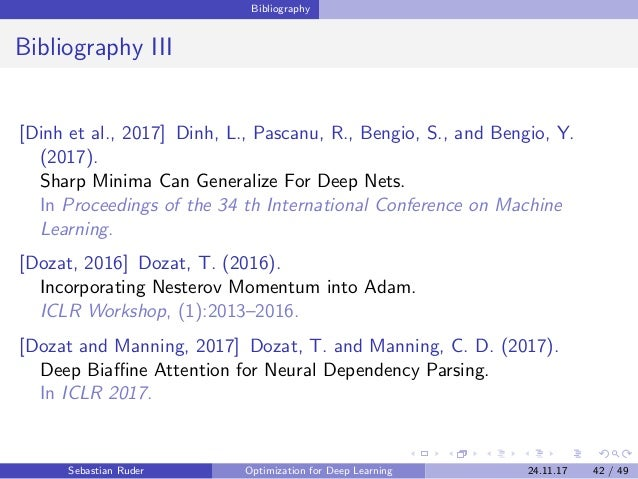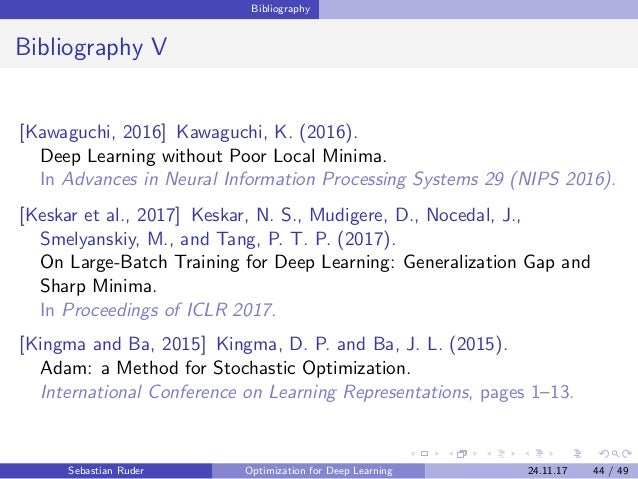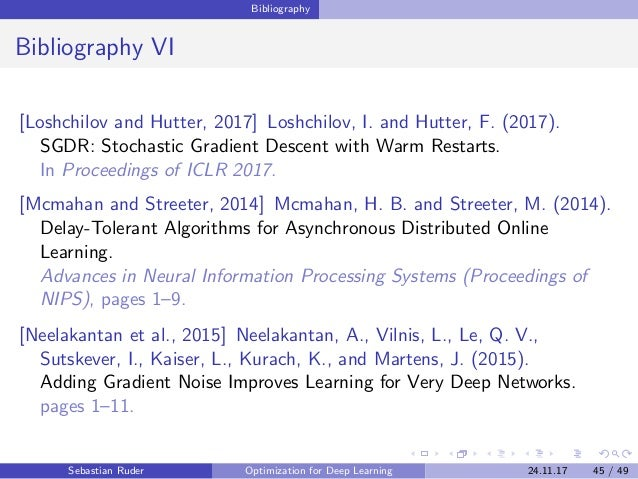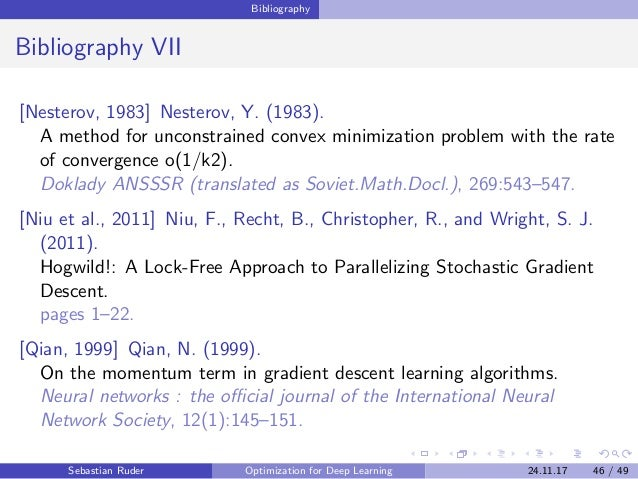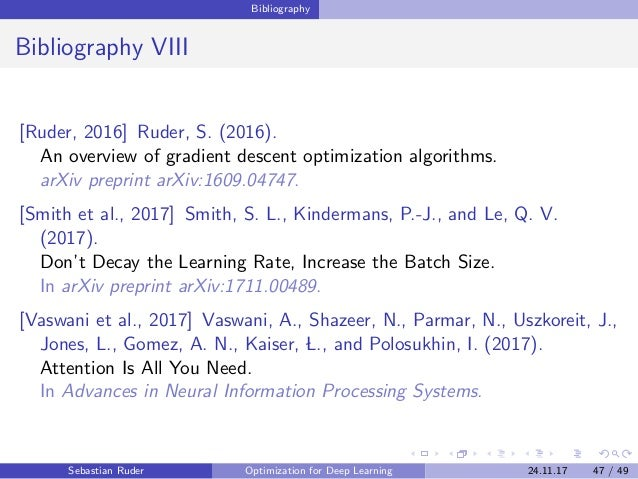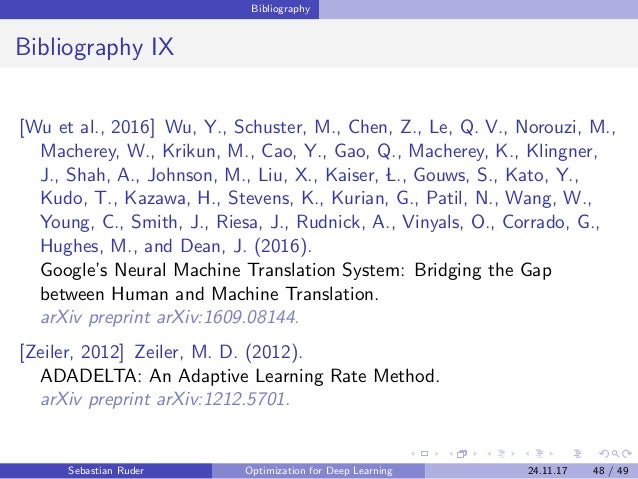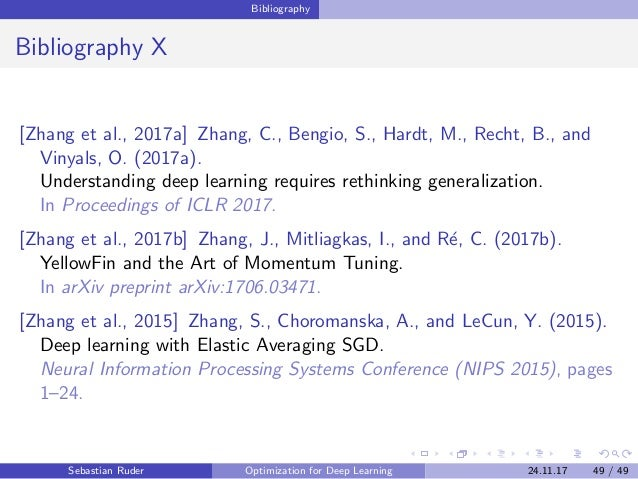Upcoming SlideShare
Loading in …5
×

# Optimization for Deep Learning

Talk on Optimization for Deep Learning, which gives an overview of gradient descent optimization algorithms and highlights some current research directions.

• Full Name
Comment goes here.

Are you sure you want to Yes No
Your message goes here• Be the first to comment

### Optimization for Deep Learning

1. 1. Optimization for Deep Learning Sebastian Ruder PhD Candidate, INSIGHT Research Centre, NUIG Research Scientist, AYLIEN @seb ruder Advanced Topics in Computational Intelligence Dublin Institute of Technology 24.11.17 Sebastian Ruder Optimization for Deep Learning 24.11.17 1 / 49
2. 2. Agenda 1 Introduction 2 Gradient descent variants 3 Challenges 4 Gradient descent optimization algorithms 5 Parallelizing and distributing SGD 6 Additional strategies for optimizing SGD 7 Outlook Sebastian Ruder Optimization for Deep Learning 24.11.17 2 / 49
3. 3. Introduction Introduction Gradient descent is a way to minimize an objective function J(θ) θ ∈ Rd : model parameters η: learning rate θJ(θ): gradient of the objective function with regard to the parameters Updates parameters in opposite direction of gradient. Update equation: θ = θ − η · θJ(θ) Figure: Optimization with gradient descent Sebastian Ruder Optimization for Deep Learning 24.11.17 3 / 49
4. 4. Gradient descent variants Gradient descent variants 1 Batch gradient descent 2 Stochastic gradient descent 3 Mini-batch gradient descent Diﬀerence: Amount of data used per update Sebastian Ruder Optimization for Deep Learning 24.11.17 4 / 49
5. 5. Gradient descent variants Batch gradient descent Batch gradient descent Computes gradient with the entire dataset. Update equation: θ = θ − η · θJ(θ) for i in range(nb_epochs ): params_grad = evaluate_gradient ( loss_function , data , params) params = params - learning_rate * params_grad Listing 1: Code for batch gradient descent update Sebastian Ruder Optimization for Deep Learning 24.11.17 5 / 49
6. 6. Gradient descent variants Batch gradient descent Pros: Guaranteed to converge to global minimum for convex error surfaces and to a local minimum for non-convex surfaces. Cons: Very slow. Intractable for datasets that do not ﬁt in memory. No online learning. Sebastian Ruder Optimization for Deep Learning 24.11.17 6 / 49
7. 7. Gradient descent variants Stochastic gradient descent Stochastic gradient descent Computes update for each example x(i)y(i). Update equation: θ = θ − η · θJ(θ; x(i); y(i)) for i in range(nb_epochs ): np.random.shuffle(data) for example in data: params_grad = evaluate_gradient ( loss_function , example , params) params = params - learning_rate * params_grad Listing 2: Code for stochastic gradient descent update Sebastian Ruder Optimization for Deep Learning 24.11.17 7 / 49
8. 8. Gradient descent variants Stochastic gradient descent Pros Much faster than batch gradient descent. Allows online learning. Cons High variance updates. Figure: SGD ﬂuctuation (Source: Wikipedia) Sebastian Ruder Optimization for Deep Learning 24.11.17 8 / 49
9. 9. Gradient descent variants Stochastic gradient descent Batch gradient descent vs. SGD ﬂuctuation Figure: Batch gradient descent vs. SGD ﬂuctuation (Source: wikidocs.net) SGD shows same convergence behaviour as batch gradient descent if learning rate is slowly decreased (annealed) over time. Sebastian Ruder Optimization for Deep Learning 24.11.17 9 / 49
10. 10. Gradient descent variants Mini-batch gradient descent Mini-batch gradient descent Performs update for every mini-batch of n examples. Update equation: θ = θ − η · θJ(θ; x(i:i+n); y(i:i+n)) for i in range(nb_epochs ): np.random.shuffle(data) for batch in get_batches(data , batch_size =50): params_grad = evaluate_gradient ( loss_function , batch , params) params = params - learning_rate * params_grad Listing 3: Code for mini-batch gradient descent update Sebastian Ruder Optimization for Deep Learning 24.11.17 10 / 49
11. 11. Gradient descent variants Mini-batch gradient descent Pros Reduces variance of updates. Can exploit matrix multiplication primitives. Cons Mini-batch size is a hyperparameter. Common sizes are 50-256. Typically the algorithm of choice. Usually referred to as SGD even when mini-batches are used. Sebastian Ruder Optimization for Deep Learning 24.11.17 11 / 49
12. 12. Gradient descent variants Mini-batch gradient descent Method Accuracy Update Speed Memory Usage Online Learning Batch gradient descent Good Slow High No Stochastic gradient descent Good (with annealing) High Low Yes Mini-batch gradient descent Good Medium Medium Yes Table: Comparison of trade-oﬀs of gradient descent variants Sebastian Ruder Optimization for Deep Learning 24.11.17 12 / 49
13. 13. Challenges Challenges Choosing a learning rate. Deﬁning an annealing schedule. Updating features to diﬀerent extent. Avoiding suboptimal minima. Sebastian Ruder Optimization for Deep Learning 24.11.17 13 / 49
14. 14. Gradient descent optimization algorithms Gradient descent optimization algorithms 1 Momentum 2 Nesterov accelerated gradient 3 Adagrad 4 Adadelta 5 RMSprop 6 Adam 7 Adam extensions Sebastian Ruder Optimization for Deep Learning 24.11.17 14 / 49
15. 15. Gradient descent optimization algorithms Momentum Momentum SGD has trouble navigating ravines. Momentum [Qian, 1999] helps SGD accelerate. Adds a fraction γ of the update vector of the past step vt−1 to current update vector vt. Momentum term γ is usually set to 0.9. vt = γvt−1 + η θJ(θ) θ = θ − vt (1) (a) SGD without momentum (b) SGD with momentum Figure: Source: Genevieve B. Orr Sebastian Ruder Optimization for Deep Learning 24.11.17 15 / 49
16. 16. Gradient descent optimization algorithms Momentum Reduces updates for dimensions whose gradients change directions. Increases updates for dimensions whose gradients point in the same directions. Figure: Optimization with momentum (Source: distill.pub) Sebastian Ruder Optimization for Deep Learning 24.11.17 16 / 49
17. 17. Gradient descent optimization algorithms Nesterov accelerated gradient Nesterov accelerated gradient Momentum blindly accelerates down slopes: First computes gradient, then makes a big jump. Nesterov accelerated gradient (NAG) [Nesterov, 1983] ﬁrst makes a big jump in the direction of the previous accumulated gradient θ − γvt−1. Then measures where it ends up and makes a correction, resulting in the complete update vector. vt = γ vt−1 + η θJ(θ − γvt−1) θ = θ − vt (2) Figure: Nesterov update (Source: G. Hinton’s lecture 6c) Sebastian Ruder Optimization for Deep Learning 24.11.17 17 / 49
18. 18. Gradient descent optimization algorithms Adagrad Adagrad Previous methods: Same learning rate η for all parameters θ. Adagrad [Duchi et al., 2011] adapts the learning rate to the parameters (large updates for infrequent parameters, small updates for frequent parameters). SGD update: θt+1 = θt − η · gt gt = θt J(θt) Adagrad divides the learning rate by the square root of the sum of squares of historic gradients. Adagrad update: θt+1 = θt − η √ Gt + gt (3) Gt ∈ Rd×d : diagonal matrix where each diagonal element i, i is the sum of the squares of the gradients w.r.t. θi up to time step t : smoothing term to avoid division by zero : element-wise multiplication Sebastian Ruder Optimization for Deep Learning 24.11.17 18 / 49
19. 19. Gradient descent optimization algorithms Adagrad Pros Well-suited for dealing with sparse data. Signiﬁcantly improves robustness of SGD. Lesser need to manually tune learning rate. Cons Accumulates squared gradients in denominator. Causes the learning rate to shrink and become inﬁnitesimally small. Sebastian Ruder Optimization for Deep Learning 24.11.17 19 / 49
20. 20. Gradient descent optimization algorithms Adadelta Adadelta Adadelta [Zeiler, 2012] restricts the window of accumulated past gradients to a ﬁxed size. SGD update: ∆θt = −η · gt θt+1 = θt + ∆θt (4) Deﬁnes running average of squared gradients E[g2]t at time t: E[g2 ]t = γE[g2 ]t−1 + (1 − γ)g2 t (5) γ: fraction similarly to momentum term, around 0.9 Adagrad update: ∆θt = − η √ Gt + gt (6) Preliminary Adadelta update: ∆θt = − η E[g2]t + gt (7) Sebastian Ruder Optimization for Deep Learning 24.11.17 20 / 49
21. 21. Gradient descent optimization algorithms Adadelta ∆θt = − η E[g2]t + gt (8) Denominator is just root mean squared (RMS) error of gradient: ∆θt = − η RMS[g]t gt (9) Note: Hypothetical units do not match. Deﬁne running average of squared parameter updates and RMS: E[∆θ2 ]t = γE[∆θ2 ]t−1 + (1 − γ)∆θ2 t RMS[∆θ]t = E[∆θ2]t + (10) Approximate with RMS[∆θ]t−1, replace η for ﬁnal Adadelta update: ∆θt = − RMS[∆θ]t−1 RMS[g]t gt θt+1 = θt + ∆θt (11) Sebastian Ruder Optimization for Deep Learning 24.11.17 21 / 49
22. 22. Gradient descent optimization algorithms RMSprop RMSprop Developed independently from Adadelta around the same time by Geoﬀ Hinton. Also divides learning rate by a running average of squared gradients. RMSprop update: E[g2 ]t = γE[g2 ]t−1 + (1 − γ)g2 t θt+1 = θt − η E[g2]t + gt (12) γ: decay parameter; typically set to 0.9 η: learning rate; a good default value is 0.001 Sebastian Ruder Optimization for Deep Learning 24.11.17 22 / 49
23. 23. Gradient descent optimization algorithms Adam Adam Adaptive Moment Estimation (Adam) [Kingma and Ba, 2015] also stores running average of past squared gradients vt like Adadelta and RMSprop. Like Momentum, stores running average of past gradients mt. mt = β1mt−1 + (1 − β1)gt vt = β2vt−1 + (1 − β2)g2 t (13) mt: ﬁrst moment (mean) of gradients vt: second moment (uncentered variance) of gradients β1, β2: decay rates Sebastian Ruder Optimization for Deep Learning 24.11.17 23 / 49
24. 24. Gradient descent optimization algorithms Adam mt and vt are initialized as 0-vectors. For this reason, they are biased towards 0. Compute bias-corrected ﬁrst and second moment estimates: ˆmt = mt 1 − βt 1 ˆvt = vt 1 − βt 2 (14) Adam update rule: θt+1 = θt − η √ ˆvt + ˆmt (15) Sebastian Ruder Optimization for Deep Learning 24.11.17 24 / 49
25. 25. Gradient descent optimization algorithms Adam extensions Adam extensions 1 AdaMax [Kingma and Ba, 2015] Adam with ∞ norm 2 Nadam [Dozat, 2016] Adam with Nesterov accelerated gradient Sebastian Ruder Optimization for Deep Learning 24.11.17 25 / 49
26. 26. Gradient descent optimization algorithms Update equations Update equations Method Update equation SGD gt = θt J(θt) ∆θt = −η · gt θt = θt + ∆θt Momentum ∆θt = −γ vt−1 − ηgt NAG ∆θt = −γ vt−1 − η θJ(θ − γvt−1) Adagrad ∆θt = − η √ Gt + gt Adadelta ∆θt = − RMS[∆θ]t−1 RMS[g]t gt RMSprop ∆θt = − η E[g2]t + gt Adam ∆θt = − η √ ˆvt + ˆmt Table: Update equations for the gradient descent optimization algorithms. Sebastian Ruder Optimization for Deep Learning 24.11.17 26 / 49
27. 27. Gradient descent optimization algorithms Comparison of optimizers Visualization of algorithms (a) SGD optimization on loss surface contours (b) SGD optimization on saddle point Figure: Source and full animations: Alec Radford Sebastian Ruder Optimization for Deep Learning 24.11.17 27 / 49
28. 28. Gradient descent optimization algorithms Comparison of optimizers Which optimizer to choose? Adaptive learning rate methods (Adagrad, Adadelta, RMSprop, Adam) are particularly useful for sparse features. Adagrad, Adadelta, RMSprop, and Adam work well in similar circumstances. [Kingma and Ba, 2015] show that bias-correction helps Adam slightly outperform RMSprop. Sebastian Ruder Optimization for Deep Learning 24.11.17 28 / 49
29. 29. Parallelizing and distributing SGD Parallelizing and distributing SGD 1 Hogwild! [Niu et al., 2011] Parallel SGD updates on CPU Shared memory access without parameter lock Only works for sparse input data 2 Downpour SGD [Dean et al., 2012] Multiple replicas of model on subsets of training data run in parallel Updates sent to parameter server; updates fraction of model parameters 3 Delay-tolerant Algorithms for SGD [Mcmahan and Streeter, 2014] Methods also adapt to update delays 4 TensorFlow [Abadi et al., 2015] Computation graph is split into a subgraph for every device Communication takes place using Send/Receive node pairs 5 Elastic Averaging SGD [Zhang et al., 2015] Links parameters elastically to a center variable stored by parameter server Sebastian Ruder Optimization for Deep Learning 24.11.17 29 / 49
30. 30. Additional strategies for optimizing SGD Additional strategies for optimizing SGD 1 Shuﬄing and Curriculum Learning [Bengio et al., 2009] Shuﬄe training data after every epoch to break biases Order training examples to solve progressively harder problems; infrequently used in practice 2 Batch normalization [Ioﬀe and Szegedy, 2015] Re-normalizes every mini-batch to zero mean, unit variance Must-use for computer vision 3 Early stopping “Early stopping (is) beautiful free lunch” (Geoﬀ Hinton) 4 Gradient noise [Neelakantan et al., 2015] Add Gaussian noise to gradient Makes model more robust to poor initializations Sebastian Ruder Optimization for Deep Learning 24.11.17 30 / 49
31. 31. Outlook Outlook 1 Tuned SGD vs. Adam 2 SGD with restarts 3 Learning to optimize 4 Understanding generalization in Deep Learning 5 Case studies Sebastian Ruder Optimization for Deep Learning 24.11.17 31 / 49
32. 32. Outlook Tuned SGD vs. Adam Tuned SGD vs. Adam Many recent papers use SGD with learning rate annealing. SGD with tuned learning rate and momentum is competitive with Adam [Zhang et al., 2017b]. Adam converges faster, but underperforms SGD on some tasks, e.g. Machine Translation [Wu et al., 2016]. Adam with 2 restarts and SGD-style annealing converges faster and outperforms SGD [Denkowski and Neubig, 2017]. Increasing the batch size may have the same eﬀect as decaying the learning rate [Smith et al., 2017]. Sebastian Ruder Optimization for Deep Learning 24.11.17 32 / 49
33. 33. Outlook SGD with restarts SGD with restarts At each restart, the learning rate is initialized to some value and decreases with cosine annealing [Loshchilov and Hutter, 2017]. Converges 2× to 4× faster with comparable performance. Figure: Learning rate schedules with warm restarts [Loshchilov and Hutter, 2017] Sebastian Ruder Optimization for Deep Learning 24.11.17 33 / 49
34. 34. Outlook SGD with restarts Snapshot ensembles 1 Train model until convergence with cosine annealing schedule. 2 Save model parameters. 3 Perform warm restart and repeat steps 1-3 M times. 4 Ensemble saved models. Figure: SGD vs. snapshot ensemble [Huang et al., 2017] Sebastian Ruder Optimization for Deep Learning 24.11.17 34 / 49
35. 35. Outlook Learning to optimize Learning to optimize Rather than manually deﬁning an update rule, learn it. Update rules outperform existing optimizers and transfer across tasks. Figure: Neural Optimizer Search [Bello et al., 2017] Sebastian Ruder Optimization for Deep Learning 24.11.17 35 / 49
36. 36. Outlook Learning to optimize Discovered update rules PowerSign: αf (t)∗sign(g)∗sign(m) ∗ g (16) α: often e or 2 f : either 1 or a decay function of the training step t m: moving average of gradients Scales update by αf (t) or 1/αf (t) depending on whether the direction of the gradient and its running average agree. AddSign: (α + f (t) ∗ sign(g) ∗ sign(m)) ∗ g (17) α: often 1 or 2 Scales update by α + f (t) or α − f (t). Sebastian Ruder Optimization for Deep Learning 24.11.17 36 / 49
37. 37. Outlook Understanding generalization in Deep Learning Understanding generalization in Deep Learning Optimization is closely tied to generalization. The number of possible local minima grows exponentially with the number of parameters [Kawaguchi, 2016]. Diﬀerent local minima generalize to diﬀerent extents. Recent insights in understanding generalization: Neural networks can completely memorize random inputs [Zhang et al., 2017a]. Sharp minima found by batch gradient descent have high generalization error [Keskar et al., 2017]. Local minima that generalize well can be made arbitrarily sharp [Dinh et al., 2017]. Several submissions at ICLR 2018 on understanding generalization. Sebastian Ruder Optimization for Deep Learning 24.11.17 37 / 49
38. 38. Outlook Case studies Case studies Deep Biaﬃne Attention for Neural Dependency Parsing [Dozat and Manning, 2017] Adam with β1 = 0.9, β2 = 0.9 Report large positive impact on ﬁnal performance of lowering β2 Attention is All You Need [Vaswani et al., 2017] Adam with β1 = 0.9, β2 = 0.98, = 10−9 , learning rate η η = d−0.5 model · min(step num−0.5 , step num · warmup steps−1.5 ) warmup steps = 4000 Sebastian Ruder Optimization for Deep Learning 24.11.17 38 / 49
39. 39. Outlook Case studies Thank you for attention! For more details and derivations of the gradient descent optimization algorithms, refer to [Ruder, 2016]. Sebastian Ruder Optimization for Deep Learning 24.11.17 39 / 49
40. 40. Bibliography Bibliography I [Abadi et al., 2015] Abadi, M., Agarwal, A., Barham, P., Brevdo, E., Chen, Z., Citro, C., Corrado, G., Davis, A., Dean, J., Devin, M., Ghemawat, S., Goodfellow, I., Harp, A., Irving, G., Isard, M., Jia, Y., Kaiser, L., Kudlur, M., Levenberg, J., Man, D., Monga, R., Moore, S., Murray, D., Shlens, J., Steiner, B., Sutskever, I., Tucker, P., Vanhoucke, V., Vasudevan, V., Vinyals, O., Warden, P., Wicke, M., Yu, Y., and Zheng, X. (2015). TensorFlow: Large-Scale Machine Learning on Heterogeneous Distributed Systems. [Bello et al., 2017] Bello, I., Zoph, B., Vasudevan, V., and Le, Q. V. (2017). Neural Optimizer Search with Reinforcement Learning. In Proceedings of the 34th International Conference on Machine Learning. Sebastian Ruder Optimization for Deep Learning 24.11.17 40 / 49
41. 41. Bibliography Bibliography II [Bengio et al., 2009] Bengio, Y., Louradour, J., Collobert, R., and Weston, J. (2009). Curriculum learning. Proceedings of the 26th annual international conference on machine learning, pages 41–48. [Dean et al., 2012] Dean, J., Corrado, G. S., Monga, R., Chen, K., Devin, M., Le, Q. V., Mao, M. Z., Ranzato, M. A., Senior, A., Tucker, P., Yang, K., and Ng, A. Y. (2012). Large Scale Distributed Deep Networks. NIPS 2012: Neural Information Processing Systems, pages 1–11. [Denkowski and Neubig, 2017] Denkowski, M. and Neubig, G. (2017). Stronger Baselines for Trustable Results in Neural Machine Translation. In Workshop on Neural Machine Translation (WNMT). Sebastian Ruder Optimization for Deep Learning 24.11.17 41 / 49
42. 42. Bibliography Bibliography III [Dinh et al., 2017] Dinh, L., Pascanu, R., Bengio, S., and Bengio, Y. (2017). Sharp Minima Can Generalize For Deep Nets. In Proceedings of the 34 th International Conference on Machine Learning. [Dozat, 2016] Dozat, T. (2016). Incorporating Nesterov Momentum into Adam. ICLR Workshop, (1):2013–2016. [Dozat and Manning, 2017] Dozat, T. and Manning, C. D. (2017). Deep Biaﬃne Attention for Neural Dependency Parsing. In ICLR 2017. Sebastian Ruder Optimization for Deep Learning 24.11.17 42 / 49
43. 43. Bibliography Bibliography IV [Duchi et al., 2011] Duchi, J., Hazan, E., and Singer, Y. (2011). Adaptive Subgradient Methods for Online Learning and Stochastic Optimization. Journal of Machine Learning Research, 12:2121–2159. [Huang et al., 2017] Huang, G., Li, Y., Pleiss, G., Liu, Z., Hopcroft, J. E., and Weinberger, K. Q. (2017). Snapshot Ensembles: Train 1, get M for free. In Proceedings of ICLR 2017. [Ioﬀe and Szegedy, 2015] Ioﬀe, S. and Szegedy, C. (2015). Batch Normalization: Accelerating Deep Network Training by Reducing Internal Covariate Shift. arXiv preprint arXiv:1502.03167v3. Sebastian Ruder Optimization for Deep Learning 24.11.17 43 / 49
44. 44. Bibliography Bibliography V [Kawaguchi, 2016] Kawaguchi, K. (2016). Deep Learning without Poor Local Minima. In Advances in Neural Information Processing Systems 29 (NIPS 2016). [Keskar et al., 2017] Keskar, N. S., Mudigere, D., Nocedal, J., Smelyanskiy, M., and Tang, P. T. P. (2017). On Large-Batch Training for Deep Learning: Generalization Gap and Sharp Minima. In Proceedings of ICLR 2017. [Kingma and Ba, 2015] Kingma, D. P. and Ba, J. L. (2015). Adam: a Method for Stochastic Optimization. International Conference on Learning Representations, pages 1–13. Sebastian Ruder Optimization for Deep Learning 24.11.17 44 / 49
45. 45. Bibliography Bibliography VI [Loshchilov and Hutter, 2017] Loshchilov, I. and Hutter, F. (2017). SGDR: Stochastic Gradient Descent with Warm Restarts. In Proceedings of ICLR 2017. [Mcmahan and Streeter, 2014] Mcmahan, H. B. and Streeter, M. (2014). Delay-Tolerant Algorithms for Asynchronous Distributed Online Learning. Advances in Neural Information Processing Systems (Proceedings of NIPS), pages 1–9. [Neelakantan et al., 2015] Neelakantan, A., Vilnis, L., Le, Q. V., Sutskever, I., Kaiser, L., Kurach, K., and Martens, J. (2015). Adding Gradient Noise Improves Learning for Very Deep Networks. pages 1–11. Sebastian Ruder Optimization for Deep Learning 24.11.17 45 / 49
46. 46. Bibliography Bibliography VII [Nesterov, 1983] Nesterov, Y. (1983). A method for unconstrained convex minimization problem with the rate of convergence o(1/k2). Doklady ANSSSR (translated as Soviet.Math.Docl.), 269:543–547. [Niu et al., 2011] Niu, F., Recht, B., Christopher, R., and Wright, S. J. (2011). Hogwild!: A Lock-Free Approach to Parallelizing Stochastic Gradient Descent. pages 1–22. [Qian, 1999] Qian, N. (1999). On the momentum term in gradient descent learning algorithms. Neural networks : the oﬃcial journal of the International Neural Network Society, 12(1):145–151. Sebastian Ruder Optimization for Deep Learning 24.11.17 46 / 49
47. 47. Bibliography Bibliography VIII [Ruder, 2016] Ruder, S. (2016). An overview of gradient descent optimization algorithms. arXiv preprint arXiv:1609.04747. [Smith et al., 2017] Smith, S. L., Kindermans, P.-J., and Le, Q. V. (2017). Don’t Decay the Learning Rate, Increase the Batch Size. In arXiv preprint arXiv:1711.00489. [Vaswani et al., 2017] Vaswani, A., Shazeer, N., Parmar, N., Uszkoreit, J., Jones, L., Gomez, A. N., Kaiser, L., and Polosukhin, I. (2017). Attention Is All You Need. In Advances in Neural Information Processing Systems. Sebastian Ruder Optimization for Deep Learning 24.11.17 47 / 49
48. 48. Bibliography Bibliography IX [Wu et al., 2016] Wu, Y., Schuster, M., Chen, Z., Le, Q. V., Norouzi, M., Macherey, W., Krikun, M., Cao, Y., Gao, Q., Macherey, K., Klingner, J., Shah, A., Johnson, M., Liu, X., Kaiser, L., Gouws, S., Kato, Y., Kudo, T., Kazawa, H., Stevens, K., Kurian, G., Patil, N., Wang, W., Young, C., Smith, J., Riesa, J., Rudnick, A., Vinyals, O., Corrado, G., Hughes, M., and Dean, J. (2016). Google’s Neural Machine Translation System: Bridging the Gap between Human and Machine Translation. arXiv preprint arXiv:1609.08144. [Zeiler, 2012] Zeiler, M. D. (2012). ADADELTA: An Adaptive Learning Rate Method. arXiv preprint arXiv:1212.5701. Sebastian Ruder Optimization for Deep Learning 24.11.17 48 / 49
49. 49. Bibliography Bibliography X [Zhang et al., 2017a] Zhang, C., Bengio, S., Hardt, M., Recht, B., and Vinyals, O. (2017a). Understanding deep learning requires rethinking generalization. In Proceedings of ICLR 2017. [Zhang et al., 2017b] Zhang, J., Mitliagkas, I., and R´e, C. (2017b). YellowFin and the Art of Momentum Tuning. In arXiv preprint arXiv:1706.03471. [Zhang et al., 2015] Zhang, S., Choromanska, A., and LeCun, Y. (2015). Deep learning with Elastic Averaging SGD. Neural Information Processing Systems Conference (NIPS 2015), pages 1–24. Sebastian Ruder Optimization for Deep Learning 24.11.17 49 / 49